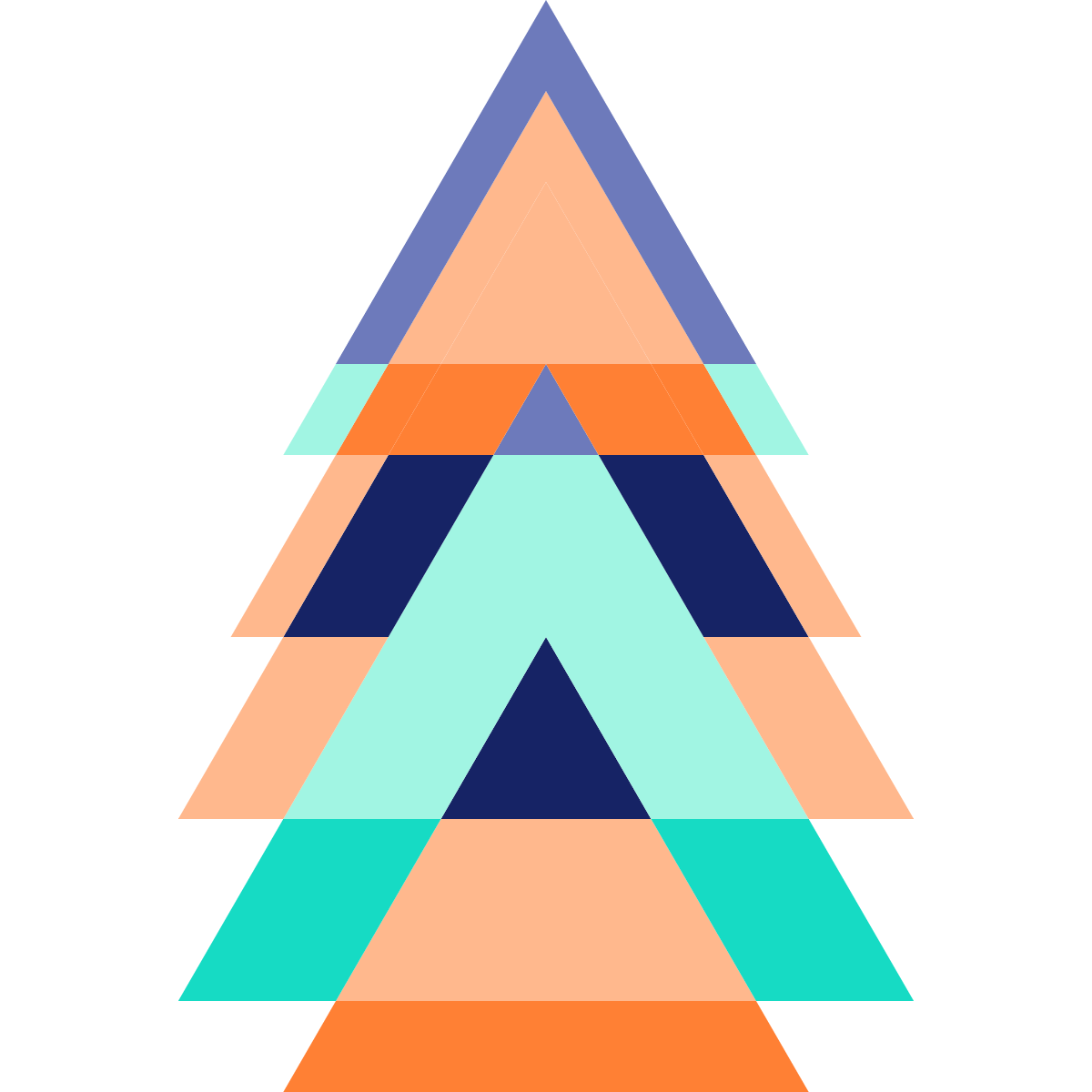# Iteration without for, foreach or while

A 3 minute read written by
Simen Endsjø
07.12.2019

Iteration is part of the bread and butter of any programming language, and this is the case in functional programming just as much as in imperative programming, but how is it possible to iterate without `for`, `foreach` or `while`?

While many functional programming languages includes regular looping constructs, this is not the preferred method. The functional approach uses recursion, but we'll rely on higher level functions like `fold` most of the time, which is implemented in terms of the lower-level recursion.

The imperative approach using a mutable variable:

``````let sum xs =
let mutable tot = 0
for x in xs do
tot <- tot + x
tot
sum [1; 10; 100]``````
``````val sum : xs:seq<int> -> int
val it : int = 111``````

Using recursion:

``````let sum xs =
let rec go tot =
function
| [] -> tot
| x::xs -> go (tot + x) xs
go 0 xs

sum [1; 10; 100]``````
``````val sum : xs:int list -> int
val it : int = 111``````

Using a fold:

``````let sum xs = Seq.fold (fun s x -> s + x) 0 xs

let a = sum [1; 10; 100]

// Or just
let sum' = Seq.fold (+) 0
let b = sum' [2; 20; 200]

// Scan returns intermediate results
let c = List.scan (+) 0 [1 .. 5]``````
``````val sum : xs:seq<int> -> int
val a : int = 111
val sum' : (int list -> int)
val b : int = 222
val c : int list = [0; 1; 3; 6; 10; 15]``````
``````val sum : xs:seq<int> -> int
val it : int = 111``````

# `fold`

`fold` (also called `reduce` or `aggregate` in some languages) is a very useful abstraction that we need examine more closely.

``Seq.fold``
``val it : (('a -> 'b -> 'a) -> 'a -> seq<'b> -> 'a) = <fun:clo@166>``

`'a` is our state, and `'b` is our element. We need a function that takes the state so far, an element from the sequence, and returns a new state. It's often used to aggregate values as with `sum`, but as long as we conform with the types, it can do pretty much everything. It might be useful to see a couple of examples.

This implements average by using a tuple containing the number of items and the running total as a tuple.

``````let avg =
Seq.fold (fun (c, t) v ->
(c+1, t+v)
) (0, 0)
>> fun (c, t) -> t/c

avg [50..100]``````
``````val avg : (int list -> int)
val it : int = 75``````

Reversing a sequence

``````let rev = Seq.fold (fun xs x -> x :: xs) []
rev [1 ..5]``````
``````val rev : (int list -> int list)
val it : int list = [5; 4; 3; 2; 1]``````

Picking the last element

``````let curry f a b = f (a, b)
let tryLast = Seq.fold (curry (snd >> Some)) None
let a = tryLast []
let b = tryLast [1; 2]``````
``````val curry : f:('a * 'b -> 'c) -> a:'a -> b:'b -> 'c
val tryLast : (int list -> int option)
val a : int option = None
val b : int option = Some 2``````

Here we count the number of equal elements in a list, and returns a new list with a tuple containing the item and count ordered by the count.

``````module Option =
let getOr x = function | Some v -> v | None -> x

let byCount =
Seq.fold (fun s k ->
Map.tryFind k s
|> Option.map ((+) 1)
|> Option.getOr 1
|> fun v -> Map.add k v s
) Map.empty
>> Map.toSeq
>> Seq.sortByDescending snd
>> Seq.toList

byCount  [1; 2; 1; 1; 1; 2; 3]``````
``````module Option = begin
val getOr : x:'a -> _arg1:'a option -> 'a
end
val byCount : (int list -> (int * int) list)
val it : (int * int) list = [(1, 4); (2, 2); (3, 1)]``````

The usecases are many. Whenever you need to work on a sequence of elements, you can usually solve it using `fold`, though it does take some practice both to read them, notice that you can use it, and write it. But by the time you've mastered folds, or at least gotten more proficient with them, you'll miss them when you only have loops available.